# 线性可分性与感知机策略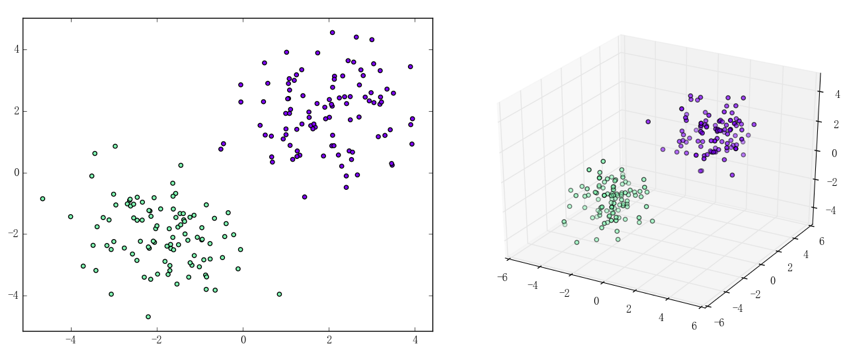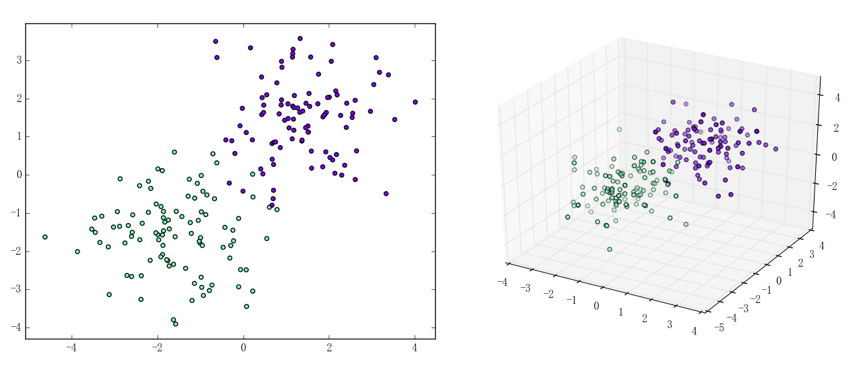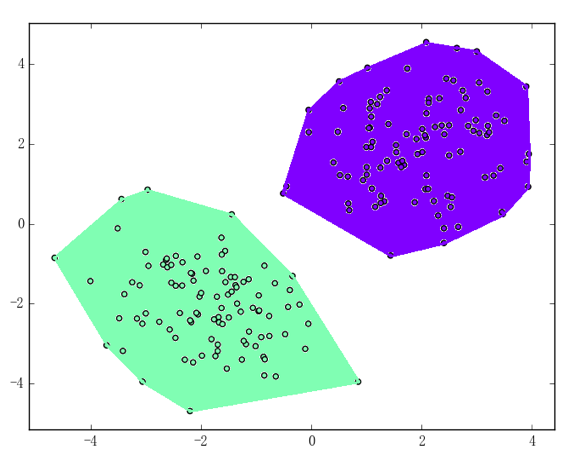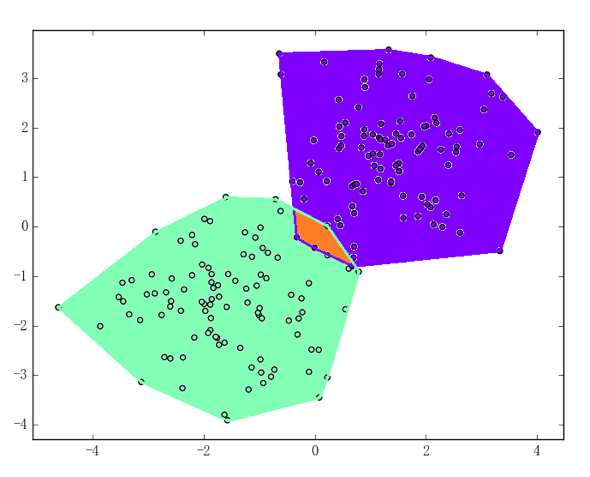# 感知机算法

1. 输入：训练数据集$D = \{\left( x_{1},y_{1} \right),\ldots,\left( x_{N},y_{N} \right)\}$、迭代次数 M、学习速率$\eta$，其中：
2. 过程
1. 初始化参数：
2. $j = 1,\ldots,M$
1. $E = \varnothing$（亦即没有误分类的样本点）则退出循环体
2. 否则，任取$E$中的一个样本点$(x_{i},y_{i})$并利用它更新参数：
3. 输出：感知机模型$g\left( x \right) = \text{sign}\left( f\left( x \right) \right) = \text{sign}\left( w \cdot x + b \right)$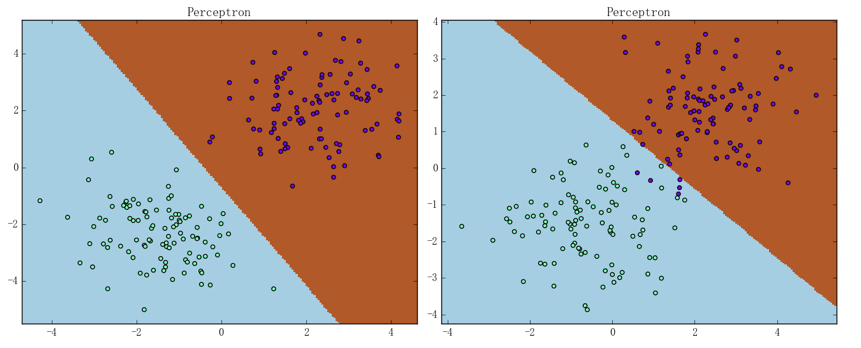# 感知机算法的对偶形式

1. 输入：训练数据集$D = \{\left( x_{1},y_{1} \right),\ldots,\left( x_{N},y_{N} \right)\}$、迭代次数 M、学习速率$\eta$，其中：
2. 过程
1. 初始化参数：
2. $j = 1,\ldots,M$
1. $E = \varnothing$（亦即没有误分类的样本点）则退出循环体
2. 否则，任取$E$中的一个样本点$(x_{i},y_{i})$并利用其下标更新参数：
3. 输出：感知机模型$g\left( x \right) = \text{sign}\left( f\left( x \right) \right) = sign\left( \sum_{k = 1}^{N}{\alpha_{k}y_{k}\left( x_{k} \cdot x_{i} + 1 \right)} \right)$